# VII Choices Among Risky Portfolios 1 Choices Among

• Slides: 23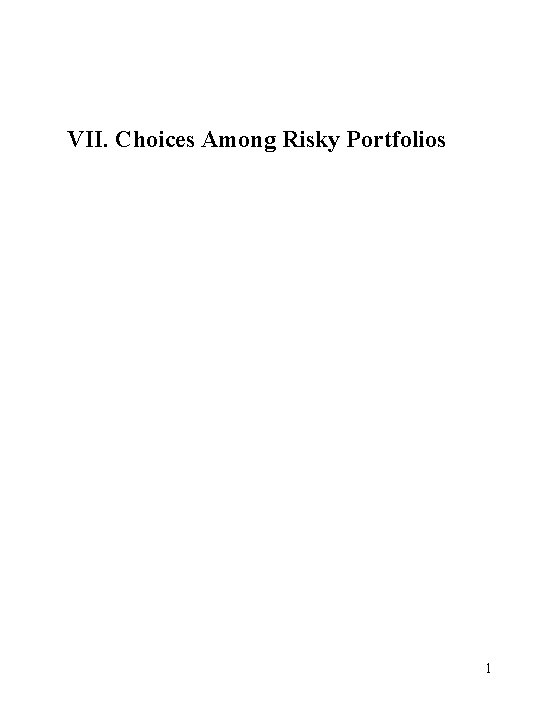VII. Choices Among Risky Portfolios 1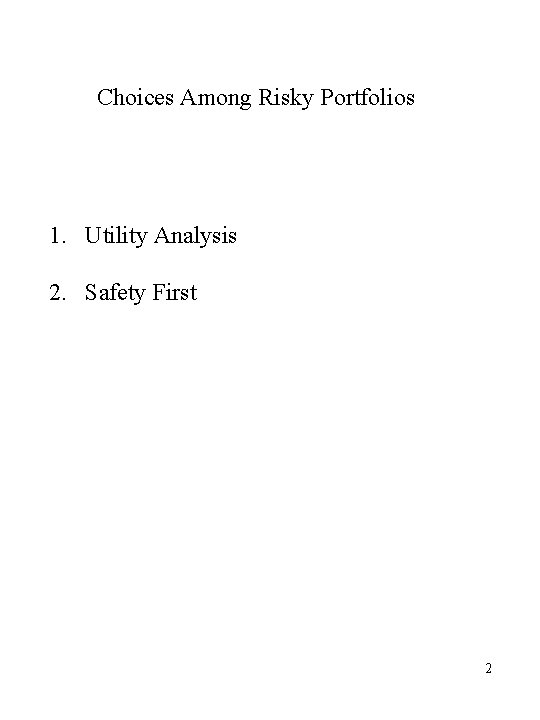Choices Among Risky Portfolios 1. Utility Analysis 2. Safety First 2Utility Analysis Choice among risky portfolios depends on risk return trade off More formally depends on maximizing value to me or utility of outcomes Utility functions are a mathematical way of determining the value of different choices to the investor 3Properties we believe utility functions for most individual should have 1. Prefer more to less – non satiation 2. Require compensation for taking risk Risk Aversion Additional Qualities to Consider 3. More of less dollars at risk as wealthier 4. Larger or smaller percentage of wealth at risk as wealthier 45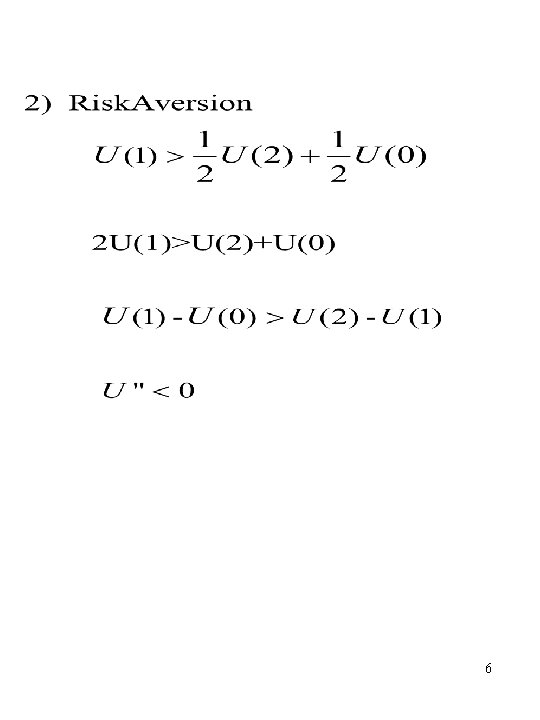67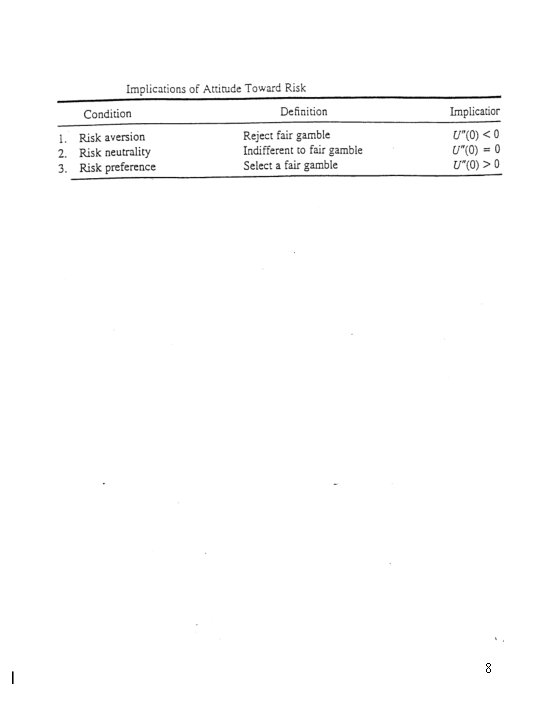8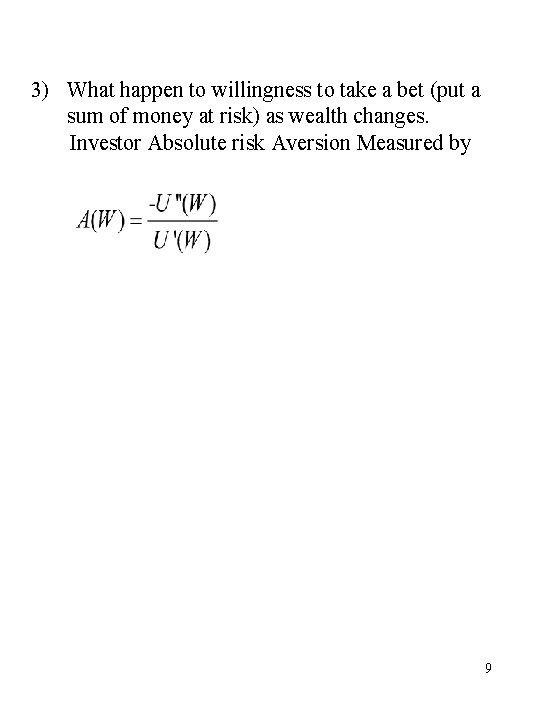3) What happen to willingness to take a bet (put a sum of money at risk) as wealth changes. Investor Absolute risk Aversion Measured by 9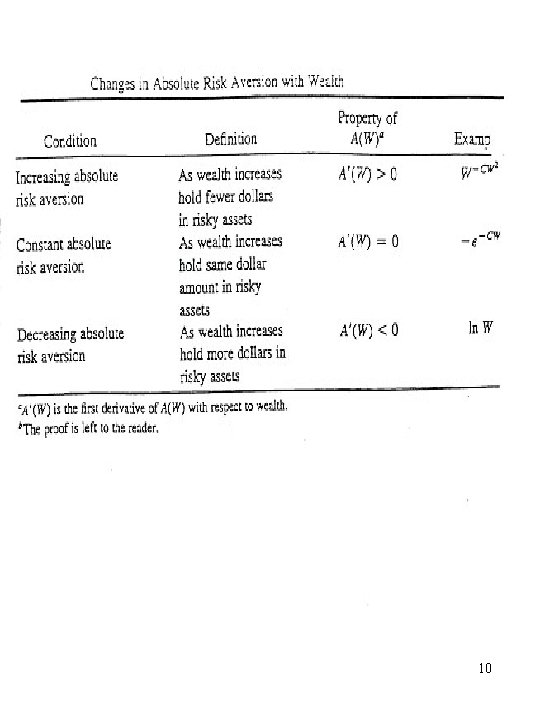10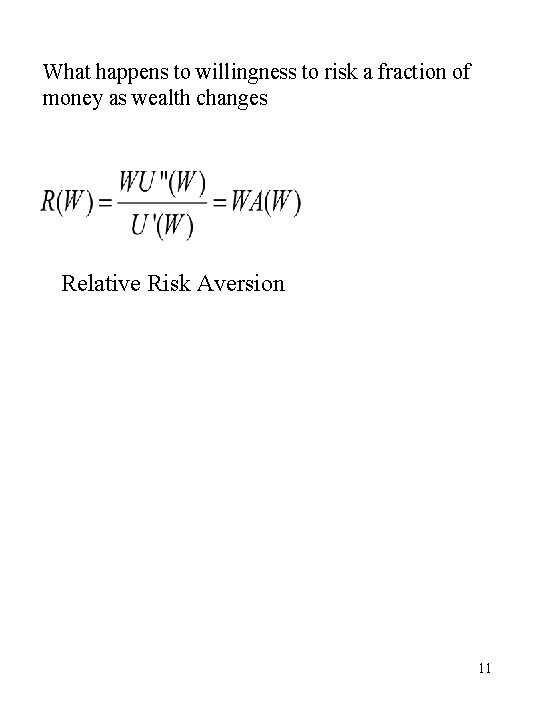What happens to willingness to risk a fraction of money as wealth changes Relative Risk Aversion 1112What do we know – most individuals exhibit 1. Non Satiation 2. Risk Aversion 3. Decreasing Absolute Risk Aversion 4. Either Constant or decreasing relative risk aversion 13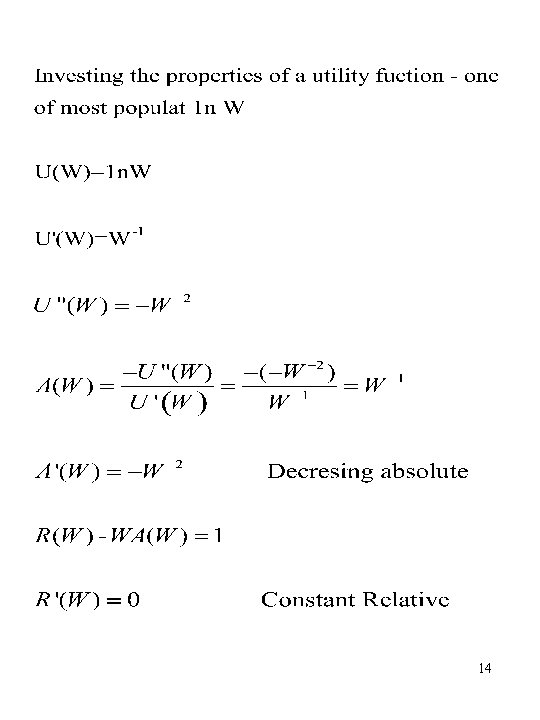14Other Portfolios Selection Criteria 1. Safety First 2. Maximize Geometric Mean 15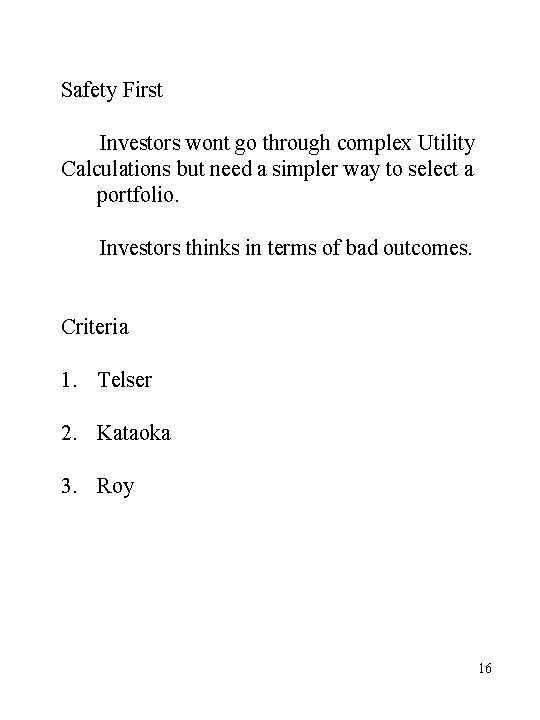Safety First Investors wont go through complex Utility Calculations but need a simpler way to select a portfolio. Investors thinks in terms of bad outcomes. Criteria 1. Telser 2. Kataoka 3. Roy 16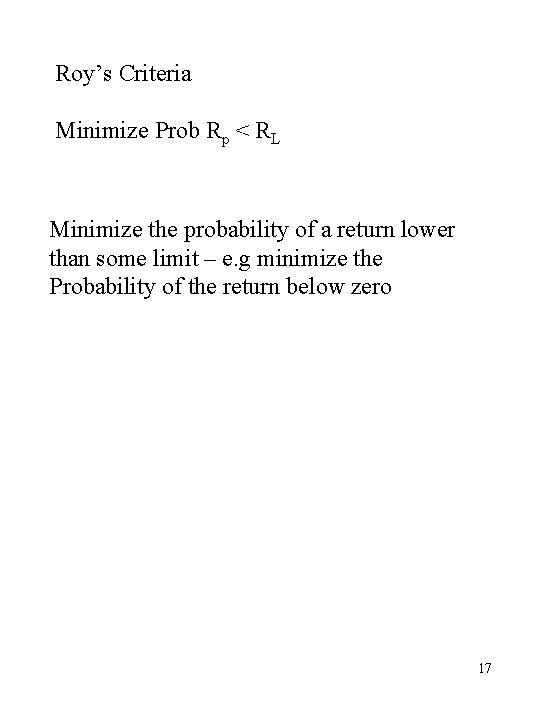Roy’s Criteria Minimize Prob Rp < RL Minimize the probability of a return lower than some limit – e. g minimize the Probability of the return below zero 1718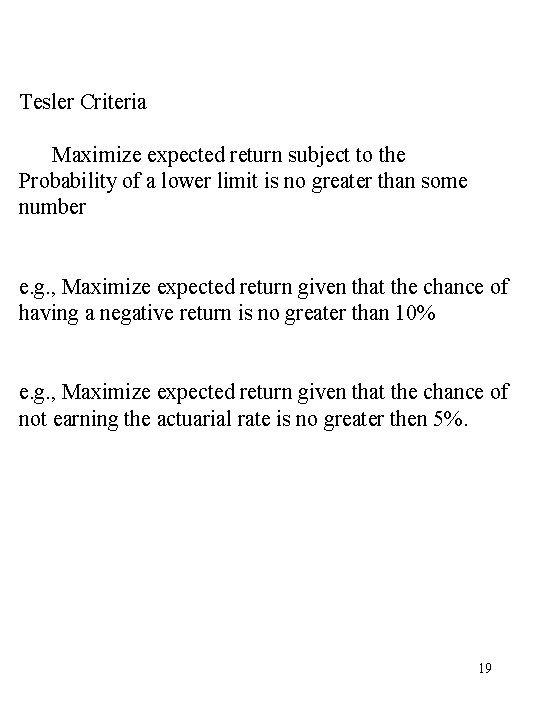Tesler Criteria Maximize expected return subject to the Probability of a lower limit is no greater than some number e. g. , Maximize expected return given that the chance of having a negative return is no greater than 10% e. g. , Maximize expected return given that the chance of not earning the actuarial rate is no greater then 5%. 1920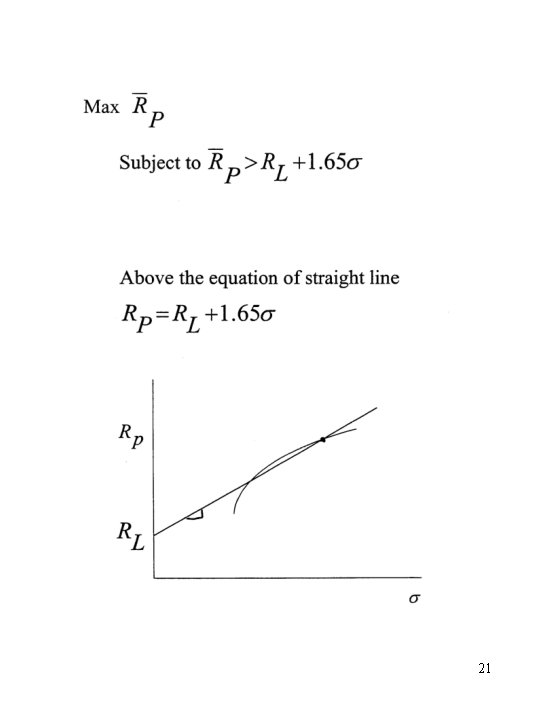21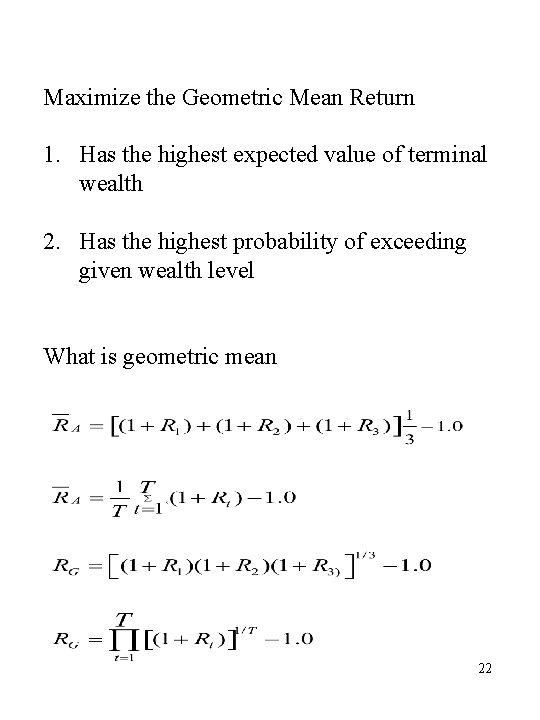Maximize the Geometric Mean Return 1. Has the highest expected value of terminal wealth 2. Has the highest probability of exceeding given wealth level What is geometric mean 22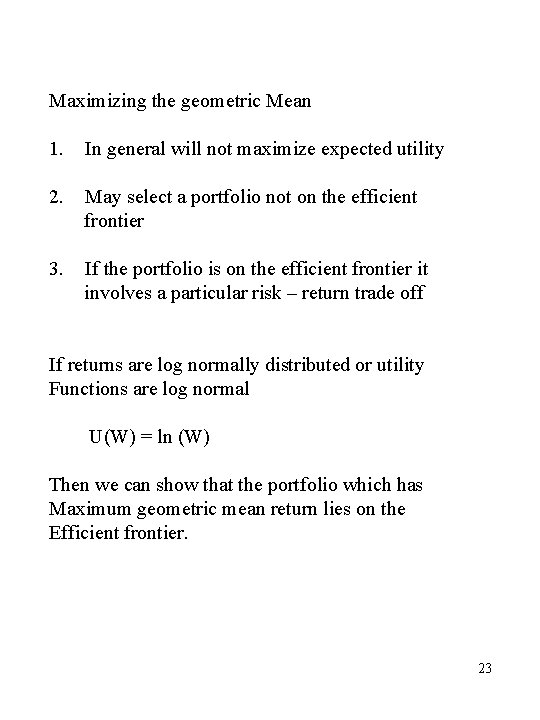Maximizing the geometric Mean 1. In general will not maximize expected utility 2. May select a portfolio not on the efficient frontier 3. If the portfolio is on the efficient frontier it involves a particular risk – return trade off If returns are log normally distributed or utility Functions are log normal U(W) = ln (W) Then we can show that the portfolio which has Maximum geometric mean return lies on the Efficient frontier. 23